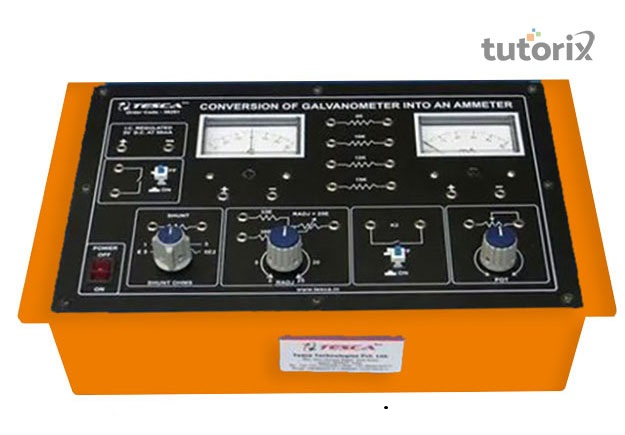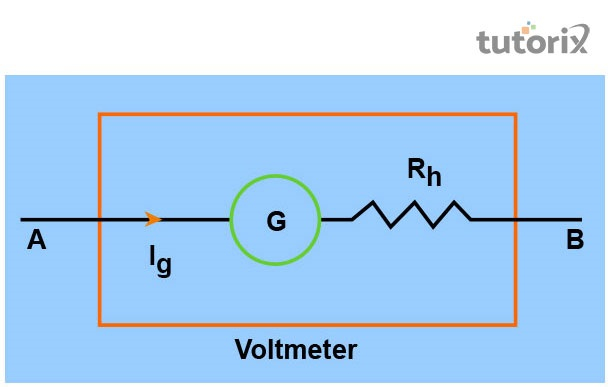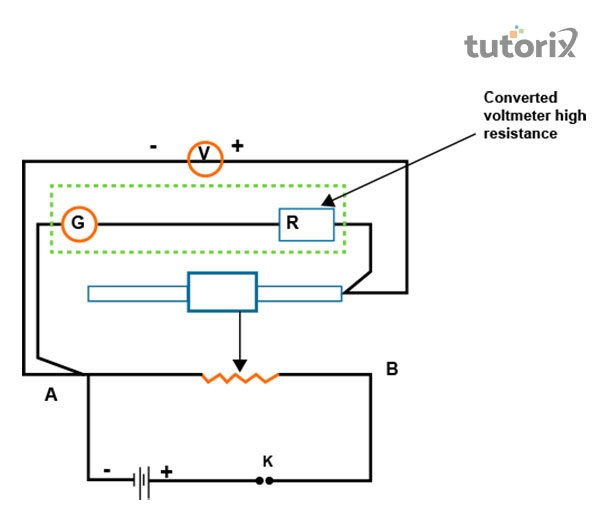# To convert the given galvanometer of known resistance and figure of merit into a voltmeter of desired range and to verify the same experiment

## Introduction

In the time of conversion of given galvanometer into a voltmeter having the desired range, it is seen that the resistance joins with the series of galvanometer. The experiment says that the determination of the resistance of a galvanometer happens through the half-deflection method and it helps to find the figure of the merit.

## Aim of conversion

There is an aim for the conversion and it says that the conversion of known resistance and figure of merit into a voltmeter of desired range is done for the verification of the same (Learncbse, 2022). Therefore, it is seen that the main aim is to convert the given galvanometer into a voltmeter of desired range and to verify the same.Figure 1: Conversion of Galvanometer to Voltmeter

## Apparatus Required

A battery, a voltmeter 0-3V, 2 boxes of resistance (200 ohms and 10,000 ohms), a galvanometer, 2 one-way keys, sandpaper and connecting wires, and a rheostat. These are the required apparatus for this process of conversion.

## Theory

Galvanometer here is an important device having the capacity to detect of weakest electric current. It is done within an electric circuit and the feature of it is provided between concave pole faces of a strong laminated horseshoe magnet. This meter visualizes the deflection during the time of passing electric current through a coil (Turusova, Nasakin & Andreeva, 2021).

It is seen that there is a directly proportional relationship between deflection and the passing current. The theory discussed that a voltmeter is an instrument and it is applied in the estimation of the electrical potential difference between the two points of an electric circuit.Figure 2: Voltmeter

## Resistance for conversion

$$\mathrm{R=V/l_g-G}$$

Here mainly refers to the range of conversion.

## Diagram and ProcedureFigure 3: Diagram

The diagram of the circuit describes the conversion of the given galvanometer of a known resistance and figure of merit into a voltmeter of desired range and it verifies the same.

## Procedure

The very first step is that one has to connect the resistance box in series combination with the galvanometer and have to take the plugs of the resistance (R). Here C is the variable terminal and A and B are the fixed terminals of the rheostat (Ncert, 2022). In this part, the galvanometer functions as a voltmeter of range of the volts (V).

Then they have to take out the plugs of the calculated R from the box. The use of a key can help to move the rheostat in a way so that the deflection of the galvanometer becomes maximum. It notes the reading of voltmeter and galvanometer and converts the reading of the galvanometer into volts. It also helps to find the difference between these two meters.

## Observation Table

deflectionThe potential difference in volts V1
1
2
3
4
5

Table 1: Observation table

### Observation and calculation

Resistance of galvanometer (G) is 60 ohm

No of division (n) = 10

Current (Jg) = nk= 0.00028A

V= range of conversion = 0-3V

Resistance in series = $\mathrm{R_s= R=V/l_g-G}$

$\mathrm{R_s= 3/0.00028 -60.7 =10653.5 \:Ohm}$

Verification $\mathrm{V_1= 3V, V_2= 2.8 V = 3-2.8/3*100 = 0.2/3*100 = 6.67}$

### Precaution

There should have to be some precautions for this stage and it says that the resistance should have to be calculated accurately. Another precaution in this matter is that for the verification of the matter there should have to use the same range conversion voltmeter (Cao et al. 2019). There should have to be the use of the current length shunt wire. It is an important part which all the connections should have to be clean, neat, and tight. It is also an important part of this conversion that the e.m.f. Of the cell or the battery will have to be constant. In this part, the ammeter that is used for the verification, should have been of the same range as that of the range of conversion. The resistance box here should have to be of the high resistance. Another aspect of conversion matter is that the use of the voltmeter that is used for the verification should have to be of the perfect range as that of the range of conversion (Notesforfree, 2022). The value of the series should have to be calculated accurately.

### Result

The result of this conversion is that as the difference between measured value and actual value of the potential is very small, the conversion remains perfect here.

## Conclusion

The idea here is the conversion of the galvanometer of a known resistance and figure of merit into a voltmeter of desired range for the verification of the matter, remains the same. The important aspect of this study is that here the series of resistance is required for the conversion process. The formula for calculation shows that V stands for the range of conversion. This process diagram has gone through the procedure that is discussed in the above points. The process also needs some precautions such as the length of the shunt should have to be perfect and it will not be too short or too long.

Q1. What is the concept of a voltmeter?

Ans: Voltmeter is an important aspect of physics and it is a device that is applied for the measurement of the potential difference between two points of the electrical circuit.

Q2. What is the principle of a galvanometer?

Ans: The main principle of this process says that it works on a principle of converting electrical energy into mechanical energy. In this matter, shunt is important and it is a small resistance and is connected in parallel with the galvanometer.

Q3. What is the process of conversion of galvanometer into voltmeter?

Ans: The process of conversion includes the connection of a high resistance in series with the help of the galvanometer coil. This is the process of conversion of galvanometer into voltmeter.

Q4. What is the consequence of converting voltmeter in series in a circuit?

Ans: It is seen that voltmeter has not the capacity of measuring potential difference within the circuit. It is because the overall resistance of the circuit increases due to the conversion.

Updated on: 13-Oct-2022

2K+ Views